Next: Tensor PICA Up: tr04cb1 Previous: Introduction

# 2 Parallel Factor Analysis (PARAFAC)

The three-way PARAFAC technique is characterised by the following generative model: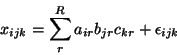(1)

(with an associated sum-of-squares loss: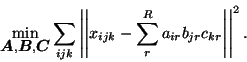(2)

Here,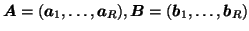and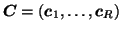denote the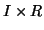,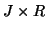and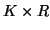matrices containing the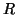different factor loadings in the temporal, spatial and subject domain as column vectors. Within this model, any solution to equation 1is a maximum likelihood solution under the assumptions of Gaussian noise.

The tri-linear model can alternatively be written in matrix notation, giving an expression for the individual 2-D subsets of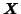[Bro, 1998]: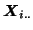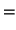(3)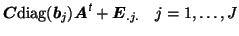(4)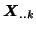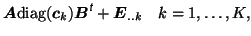(5)

where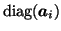denotes a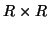diagonal matrix where the diagonal elements are taken from the elements in row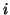of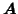(similarly for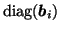and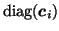). This gives rise to a set of coupled sum-of-square loss functions. Based on these, a simple way of estimating the factor matrices is to use an iterative Alternating Least Squares (ALS) approach, iterating between the least-squares estimates for one ofand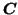separately while keeping the other two matrices fixed at their most recent estimate: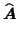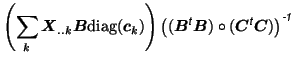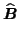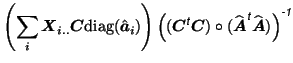(6)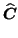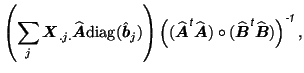where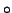denotes the direct (or element-wise) product. The ALS algorithm iteratively calculates OLS estimates for the three factor matrices. Directly fitting these so as to minimise the sum-of-squares error provides a simple way of jointly estimating the factor loadings that describe processes in the temporal, spatial and subject domain without requiring orthogonality between factor loadings in any one of the domains: the multi-way PARAFAC model, unlike PCA, does not suffer from rotational indeterminacy, i.e. a rotation of estimated factors has impact on the overall fit [Harshman and Lundy, 1984,Harshman and Lundy, 1994]. The ALS algorithm , however, can suffer from slow convergence, in particular, when a set of column vectors in one of the factor matrices is (close to being) collinear. Also, it is sensitive to specifying the correct number of factors(i.e. the number of columns inand). In order to address these issues, [Cao et al., 2000] have proposed to extend the standard PARAFAC loss function to include a diagonalisation error, such that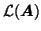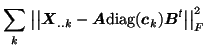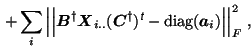(7)

(similarly for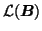and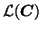). Here,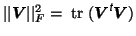denotes the Fröbenius norm and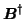denotes the pseudo-inverse of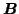. The first term corresponds to the sum-of-square loss function while the second term penalises the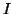differentprojection matrices. A modified ALS algorithm can be derived by iterating solutions for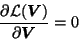with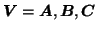. The ordinary least-squares solutions then becomes [Cao et al., 2000]: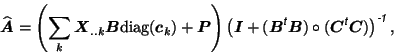where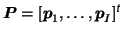and whereare column vectors formed by the elements on the main diagonal of thematrix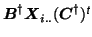(similar for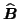and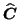). This modified ALS algorithm has been used for all later PARAFAC calculation.

It is interesting to note that the ALS approach to three-way PARAFAC does provide a unique decomposition, provided the data has appropriate 'system variation' [Harshman and Lundy, 1984,Harshman and Lundy, 1994], i.e. whenandare of full rank and there are proportional changes in the relative contribution from one factor to another in all three domains so that no two factors in any domain are collinear. In FMRI, however, we might expect the individual vectors in subject space to exhibit a significant amount of collinearity between some of them, e.g. in the case of two spatially different physiological signals, we might expect the relative contribution of the individual subjects to be very similar, so that two columns inare (close to being) collinear. The effects of collinearity of some of the factors on the ability to extract the latent structure of the data will be evaluated in section 4.Next: Tensor PICA Up: tr04cb1 Previous: Introduction
Christian Beckmann 2004-12-14